# Exchangeable Probability Distribution

Let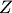be a measurable space. A probability distribution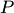on the measurable space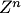of sequences of length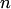, where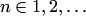, is exchangeable iffor any measurable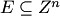and any permutation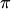of the set(i.e., if the distribution of the sequenceis invariant under any permutation of the indices).

A probability distributionon the power measurable space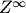is exchangeable if the marginal distribution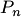ofon(defined by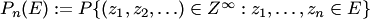for all events) is exchangeable for each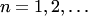(i.e., if the distribution of the sequenceis invariant under any permutation of the finite number of the indices).